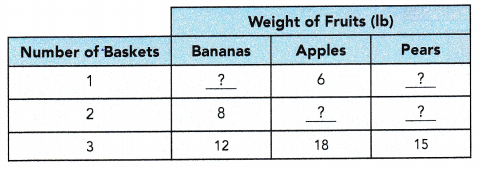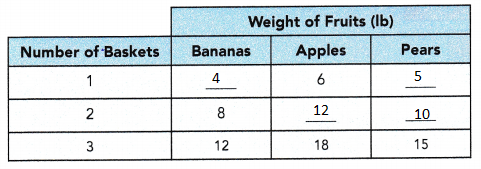# Math in Focus Grade 6 Chapter 4 Lesson 4.2 Answer Key Equivalent Ratios

Go through the Math in Focus Grade 6 Workbook Answer Key Chapter 4 Lesson 4.2 Equivalent Ratios to finish your assignments.

## Math in Focus Grade 6 Course 1 A Chapter 4 Lesson 4.2 Answer Key Equivalent Ratios

### Math in Focus Grade 6 Chapter 4 Lesson 4.2 Guided Practice Answer Key

Complete.

Question 1.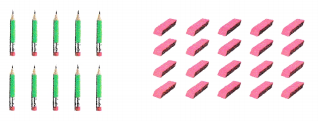The ratio of the number of pencils to the number of erasers is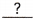:.
1 : 2
Explanation:
The ratio of the number of pencils to the number of erasers is
10 : 20 = 1 : 2

Question 2.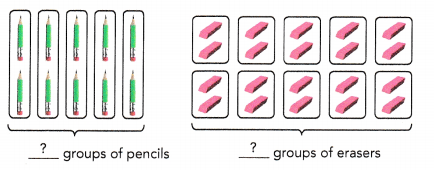The ratio of the number of groups of pencils to the number of groups of erasers is:.
1 : 2
Explanation: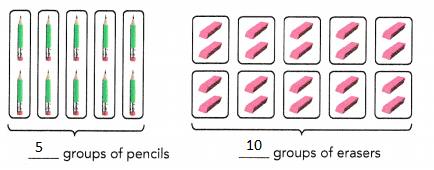The ratio of the number of groups of pencils to the number of groups of erasers is
5 : 10 = 1 : 2

Question 3.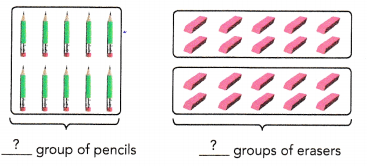The ratio of the number of groups of pencils to the number of groups of erasers is:.
1 : 2
Explanation: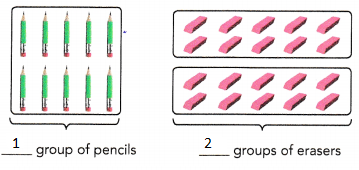The ratio of the number of groups of pencils to the number of groups of erasers is = 1 : 2

Question 4.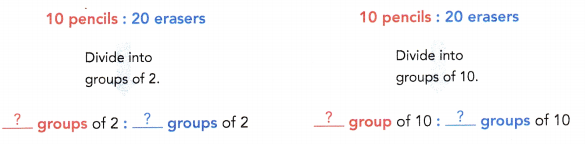The ratio:,:, and:are equivalent ratios.
1 : 2
Explanation:
The ratio 10 : 20, 5 : 10, and  1:2 are equivalent ratios.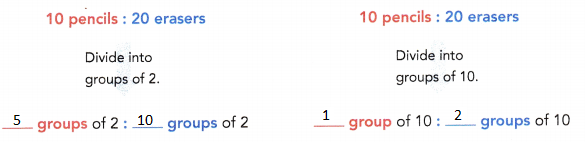Question 5.
Express the ratio 12 : 64 in simplest form.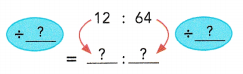3 : 16
Explanation:
the ratio 12 : 64 in simplest form.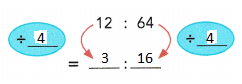Question 6.
Express the ratio 7 kg : 21 g in simplest form.
7 kg =g
7 kg : 21 g =g :g
=÷:÷=:1000 : 3
Explanation:
7 kg = 7000 g
7 kg : 21 g = 7000 g : 21 g
= 7000 ÷ 7 : 21 ÷ 7
= 1000 : 3

State whether each pair of ratios are equivalent.

Question 7.
7 : 8 and 8 : 7
NO, pair of ratios are not equivalent.
Explanation:
7 : 8 and 8 : 7 are not equivalent

Question 8.
5 : 9 and 15 : 27
YES, pair of ratios are equivalent
Explanation:
5 : 9 and 15 : 27
5 : 9 and 15 ÷ 3 : 27 ÷  3
5 : 9 and 5 : 9
5 : 9 and 15 : 27 are equivalent

Question 9.
12 : 13 and 24 : 39
NO, pair of ratios are not equivalent.
Explanation:
12 : 13 and 24 : 39
For and 12, 24 common factor is 2,
where as 13 and 39 has common factor 13 .
12 : 13 and 24 : 39 are not equivalent ratios.

Question 10.
4 : 24 and 8 : 48
YES, pair of ratios are equivalent.
Explanation:
4 : 24 and 8 : 48
4 : 24 and 8 ÷ 2 : 48 ÷ 2
4 : 24 and 8 : 48 are equivalent ratios.

Complete.

Question 11.
Use multiplication to find three ratios equivalent to 7 : 8.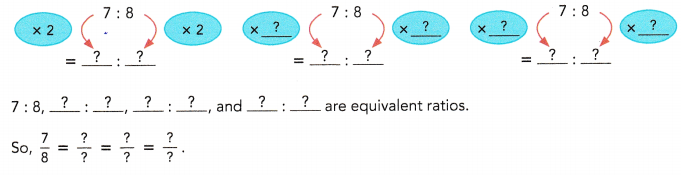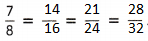Explanation: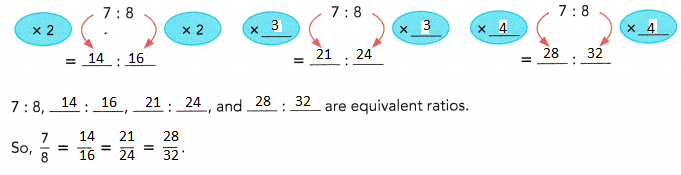Question 12.
Use division to find all the whole number ratios that are equivalent to 24 : 96.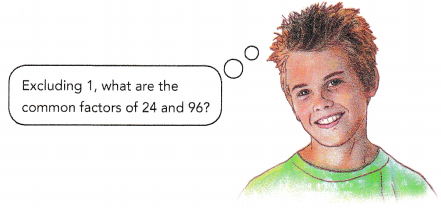24 : 96
Explanation:
24 ÷ 2 : 96 ÷ 2  => 12 : 48
24 ÷ 3 : 96 ÷ 3  => 8  : 32
24 ÷ 4 : 96 ÷ 4  => 6 : 24
24 ÷ 6 : 96 ÷ 6  => 4 : 16
24 ÷ 8 : 96 ÷ 8  => 3 : 12

Find the missing term in each pair of equivalent ratios.

Question 13.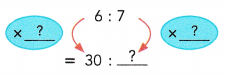30 : 35
Explanation:
6 : 7
multiply both the numbers with 5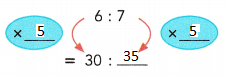Question 14.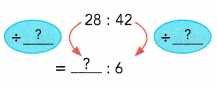4 : 6
Explanation:
4 : 6
multiply both the numbers with 7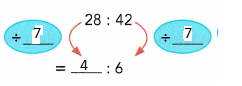Question 15.
48 : 64 =: 8
Explanation:
48 : 64
divided both the numbers with 8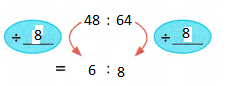Question 16.
4 : 9 = 36 :Explanation:
4 : 9
multiply both the numbers with 9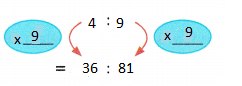Solve.

Question 17.
Selena and Drew each has a summer job. The table shows the amount of money they earn, based on the number of hours they work.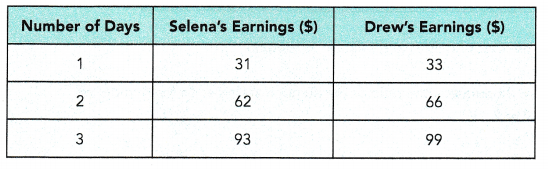a) Express the ratio of Selena’s earnings to Drew’s earnings in simplest form.
31 : 33
Explanation:
Selena and Drew each has a summer job.
for 1 day Selena earns $31 for 1 day Drew earns$33
ratio of Selena’s earnings to Drew’s earnings = 31 : 33

b) If Selena works 4 days, she will earn $. If Selena works 30 days, she will earn$.
$930 Explanation: If Selena works 4 days, she will earn$124.
for 1 day Selena earns $31 for 4 days = 31 x 4 = 124 If Selena works 30 days, she will earn$930.
31 x 30 = 930

c) If Drew works 4 days, he will earn $. If Drew works 30 days, he will earn$.
If Drew works 4 days, he will earn $132. If Drew works 30 days, he will earn$990.
Explanation:
for 1 day Drew earns \$33
for 4 days = 33 x4 = 132
for 30 days = 30 x 33 = 990

Question 18.
A school organized a paper recycling competition. The table shows the amount of oil and the amount of water saved by recycling paper.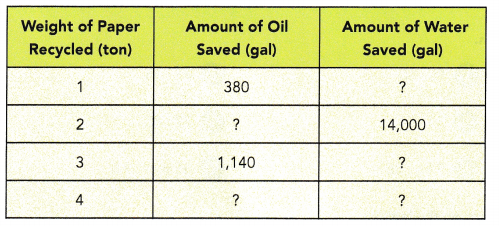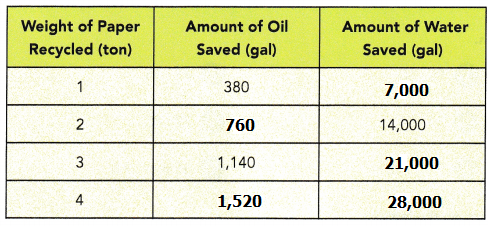a) How many gallons of water will be saved if 1 ton of paper is recycled?
7,000gal
Explanation:
for 2 tons the amount of water saved is 14,000
So, for 1 ton 14000 ÷ 2 = 7000

b) Express the ratio of the amount of oil saved to the amount of water saved in simplest form.
19 : 350
Explanation:
Weight of paper recycled for 1 ton =
Amount of oil saved : Amount of water saved
380 : 7000
= 19 : 350

c) How many gallons of oil will be saved if 2 tons of paper are recycled?
760gal
Explanation:
for 1 ton of paper recycle need 380gal of oil saved.
for 2 tons oil saved = 380 x 2 = 760

d) How many gallons of water will be saved if 3 tons of paper are recycled?
21,000gal
Explanation:
for 1 ton of paper recycle need 7,000gal of water saved.
for 2 tons water saved = 7000 x 3 = 21,000

e) How many gallons of oil and water will be saved if 4 tons of paper are recycled?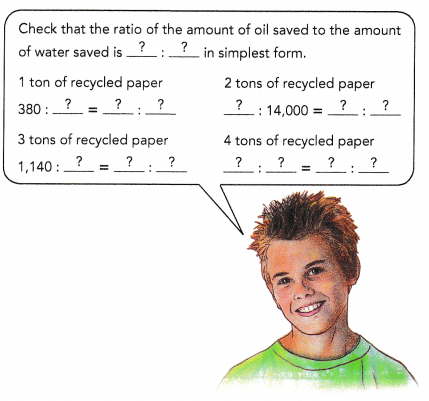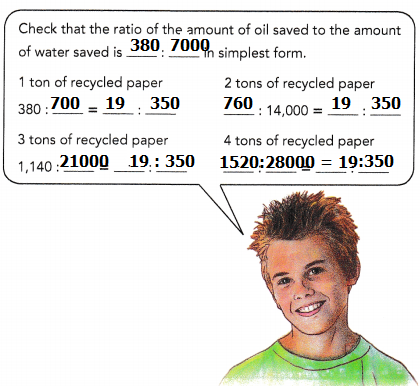Explanation:
for 1 ton of paper recycle need 7,000gal of water saved.
for 4 tons water saved = 7000 x 4 = 28,000gal
for 1 ton of paper recycle need 380gal of oil saved.
for 4 tons oil saved = 380 x 4 = 1520gal

### Math in Focus Course 1A Practice 4.2 Answer Key

Express each ratio in simplest form.

Question 1.
13 : 39
1 : 3
Explanation:
13 : 39
common factor for both the numbers is 13
so, 1 : 3

Question 2.
16 : 40
2 : 5
Explanation:
16 : 40
common factor for both the numbers is 8
so, 2 : 5

Question 3.
25 : 15
5 : 3
Explanation:
25 : 15
common factor for both the numbers is 5
so, 5 : 3

Question 4.
56 : 21
8 : 3
Explanation:
56 : 21
common factor for both the numbers is 7
so, 8 : 3

Question 5.
30 : 54
5 : 9
Explanation:
30 : 54
common factor for both the numbers is 6
so, 5 : 9

Question 6.
72 : 48
8 : 6
Explanation:
72 : 38
common factor for both the numbers is 8
so, 8 : 6

Question 7.
26 cm : 4 m
13cm : 200cm
Explanation:
26cm : 4m
convert m in cm
1m = 100 cm
4m = 400cm
26cm : 400cm
common factor for both the numbers is 2
so, 13 : 200

Question 8.
9 kg : 36 g
250g : 1g
Explanation:
9kg : 36g
convert kg to g
1kg = 100o g
9kg = 9000g
250g : 1g
common factor for both the numbers is 9
so, 250 : 1

Question 9.
35 min : 2 h
7min : 24min
Explanation:
35m : 2h
convert hr in min
1hr = 60min
2hr = 120min
35min : 120min
common factor for both the numbers is 5
so, 7 : 24

State whether each pair of ratios are equivalent.

Question 10.
11 : 17 and 17 : 11
No, pair of ratios are not equal
Explanation:
11 : 17 and 17 : 11
the given numbers are not equivalent to their ratios.

Question 11.
7 : 11 and 21 : 33
YES, pair of ratios are equal.
Explanation:
7 : 11 and 21 : 33
The common factor for both the numbers is 3
So, both are equivalent.

Question 12.
15 : 35 and 25 : 45
NO, pair of ratios are not equal.
Explanation:
15 : 35 and 25 : 45
3 : 7  and 5 : 9
The common factor for both the numbers is 5
So, both are not equivalent.

Question 13.
15 : 20 and 20 : 25
NO, pair of ratios are not equal.
Explanation:
15 : 20 and 20 : 25
3 : 4 and 4 : 5
So, both are not equivalent.

Question 14.
38 : 19 and 2 : 1
NO, pair of ratios are not equal.
Explanation:
38 : 19 and 2 : 1
2:1 and 1:2 [19 x 2 =38 and 19 x 1 = 19]
So, both are not equivalent.

Question 15.
12 : 8 and 18 : 12
YES, pair of ratios are equal.
Explanation:
12 : 8 and 18 : 12
3 : 2 and 3 : 2
So, both are equivalent.

Find the missing term in each pair of equivalent ratio.

Question 16.
7 : 9 = 49 :Explanation:
product of extremes = product of means
a:b = c:d
$$\frac{a}{b}$$ = $$\frac{c}{d}$$
a = 7, b = 9, c = 49, d = x
7 x x = 9 x 49
7x = 441
x = $$\frac{441}{7}$$
x = 63

Question 17.
12 : 5 = 144 :Explanation:
product of extremes = product of means
a:b = c:d
$$\frac{a}{b}$$ = $$\frac{c}{d}$$
a =12, b = 5, c = 144, d = x
12 x x = 5 x 144
12x = 720
x = $$\frac{720}{12}$$
x = 60

Question 18.
4 : 15 = 48 :Explanation:
product of extremes = product of means
a:b = c:d
$$\frac{a}{b}$$ = $$\frac{c}{d}$$
a = 4, b = 15, c = 48, d = x
4 x x = 15 x 48
4x = 720
x = $$\frac{720}{4}$$
x = 180

Question 19.
7 : 13 = 77 :Explanation:
product of extremes = product of means
a:b = c:d
$$\frac{a}{b}$$ = $$\frac{c}{d}$$
a = 7, b = 13, c = 77, d = x
7 x x = 13 x 77
7x = 1,001
x = $$\frac{1001}{7}$$
x = 143

Question 20.
45 : 36 =: 12
Explanation:
product of extremes = product of means
a:b = c:d
$$\frac{a}{b}$$ = $$\frac{c}{d}$$
a = 45, b = 36, c = x, d = 12
45 x 12 = 36 x x
36x = 540
x = $$\frac{540}{36}$$
x = 15

Question 21.
30 : 48 =: 8
Explanation:
product of extremes = product of means
a:b = c:d
$$\frac{a}{b}$$ = $$\frac{c}{d}$$
a = 30, b = 48, c = x, d = 8
30 x 8 = 48 x x
48x = 240
x = $$\frac{240}{48}$$
x = 5

Question 22.
72 : 84 =: 7
Explanation:
product of extremes = product of means
a:b = c:d
$$\frac{a}{b}$$ = $$\frac{c}{d}$$
a = 72, b = 84, c = x, d = 7
72 x 7 = 84 x x
504 = 84x
x = $$\frac{504}{84}$$
x = 6

Question 23.
121 : 88 =: 8
Explanation
product of extremes = product of means
a:b = c:d
$$\frac{a}{b}$$ = $$\frac{c}{d}$$
a = 121, b = 88, c = x, d = 8
121 x 8 = 88 x x
88x = 968
x = $$\frac{968}{88}$$
x = 11

Find the equivalent ratios.

Question 24.
Use multiplication to find three ratios equivalent to 8 : 12.
The three equivalent ratios of 8 : 12 are 16 : 24; 24: 36 and 32 : 48.
Explanation:
first we need to write the given ratio as fraction = 8/12
= (8 × 2)/(12 × 2)
= 16/24
= 16 : 24 (one equivalent ratio),
So, 16 : 24 is an equivalent ratio of 8 : 12.
Similarly again, we need to write the given ratio 8 : 12 as fraction to get another equivalent ratio = 8/12
= (8 × 3)/(12 × 3)
= 24/36 is another equivalent ratio.
Similarly again, we need to write the given ratio 8 : 12 as fraction to get another equivalent ratio = 8/12
= (8 × 4)/(12 × 4)
= 32/48 is another equivalent ratio.
Therefore, the three equivalent ratios of 8 : 12 are 16 : 24; 24: 36 and 32 : 48.

Question 25.
Use division to find all the whole number ratios equivalent to 168 : 56.
84 : 28; 56: 18 and 42 : 14.
Explanation:
first we need to write the given ratio as fraction = 168/56
= (168 ÷ 2)/(56 ÷ 2)
= 84/28
So, 168 : 56 is an equivalent ratio of 84 : 28.
Similarly again, we need to write the given ratio 168 : 56 as fraction to get another equivalent ratio = 168/56
= (168 ÷ 3)/(56 ÷ 3)
= 56/18
So, 168 : 56 is an equivalent ratio of 56 : 18.
Similarly again, we need to write the given ratio 168 : 56 as fraction to get another equivalent ratio = 168/56
= (168 ÷ 4)/(56 ÷ 4)
= 42/14
So, 168 : 56 is an equivalent ratio of 42 : 14.
Therefore, the two equivalent ratios of 168 : 56 are 84 : 28; 56: 18 and 42 : 14.

Copy and complete.

Question 26.
A manufacturer’s instruction states that 3 cups of cleaning agent should be diluted with 5 cups of water before use. Copy and complete the table.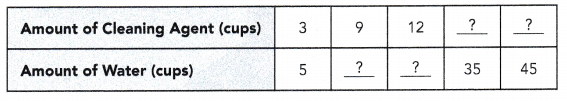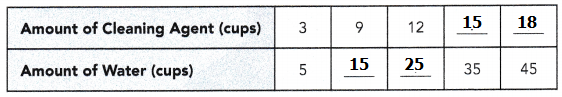Explanation:
A manufacturer’s instruction states that 3 cups of cleaning agent should be diluted with 5 cups of water before use.
ratio of cleaning agent and water = 3 : 5

Find the missing term of each pair of equivalent ratio.

Question 27.
63 : 27 = 49 :Explanation:
the product of extremes = the product of means
a:b :: c:d  = > a x d = b x c
(27 x 49) /63 = 21
63 : 27 = 49 : 21

Question 28.
81 : 18 = 36 :Explanation:
the product of extremes = the product of means
a:b :: c:d  = > a x d = b x c
(18 x 36) /81 = 648/81 = 8
81 : 18 = 36 : 8

Question 29.
24 : 96 = 5 :Explanation:
the product of extremes = the product of means
a:b :: c:d  = > a x d = b x c
(96 x 5) /24 = 480/24 = 20
24 : 96 = 5 : 20

Question 30.
72 : 24 = 15 :Explanation:
the product of means = the product of extremes
a:b :: c:d  = > a x d = b x c
(24 x 15) /72 = 360/72 = 5
72 : 24 = 15 : 5

Question 31.
60 : 144 =: 60
Explanation:
the product of means = the product of extremes
a:b :: c:d  = > a x d = b x c
(60 x 60) /144= 3600/144 = 25
60 : 144 = 25 : 60

Question 32.
125 : 80 =: 48
Explanation:
the product of extremes = the product of means
a:b :: c:d  = > a x d = b x c
(125 x 48) /80 = 6000/80 = 75
125 : 80 = 75 : 48

Question 33.
90 : 15 =: 7
Explanation:
the product of extremes = the product of means
a:b :: c:d  = > a x d = b x c
(90 x 7) /15 = 630/15 = 42
90 : 15 = 42 : 7

Question 34.
98 : 112 = 63 :Explanation:
the product of extrems = the product of means
a:b :: c:d  = > a x d = b x c
(112 x 63) /98 = 7056/98 = 72
98 : 112 = 63 : 72

Solve.

Question 35.
Judy uses 5 ounces of lemonade concentrate for every 9 ounces of orange juice concentrate to make a fruit punch.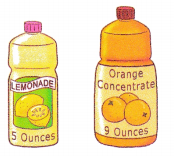a) Find the ratio of the number of ounces of orange juice concentrate to the number of ounces of lemonade concentrate she uses.
9 : 5
Explanation:
Judy uses 5 ounces of lemonade concentrate for every 9 ounces of orange juice concentrate to make a fruit punch.
The ratio of the orange juice and lemonade = 9 : 5

b) If Judy uses 36 ounces of orange juice concentrate to make the fruit punch, how many ounces of lemonade concentrate does she use?
20 oz
Explanation:
If Judy uses 36 ounces of orange juice concentrate to make the fruit punch,
how many ounces of lemonade concentrate does she use
9 : 5 = 36 : ?
the product of means = the product of extremes
a:b :: c:d  = > a x d = b x c
(36 x 5) /9 = 180/9 = 20
9 : 5 = 36 : 20

c) If Judy uses 45 ounces of lemonade concentrate to make the fruit punch, how many ounces of orange juice concentrate does she use?
81 oz
Explanation:
If Judy uses 45 ounces of lemonade concentrate to make the fruit punch,
how many ounces of orange juice concentrate does she use
9 : 5 = ? : 45
the product of means = the product of extremes
a:b :: c:d  = > a x d = b x c
(9 x 45) /5 = 405/5 = 81
9 : 5 = 81 : 45

Question 36.
In a science experiment, Farah mixed a salt solution and vinegar in the ratio 3 : 7.
a) If she used 262.8 milliliters of salt solution1 how much vinegar did she use?
183.9ml
Explanation:
The ratio of vinegar to total mixture is 7 : 10
The 10 is 3 + 7.  Out of 10 ml, 3 will be Salt and 7 will be Vinegar.
7/10 = V/262.8
1839.6 = 10V
V = 183.9 ml

b) If 0.56 liter of vinegar was used, how much salt solution did she use?
78.84 ml
Explanation:
The ratio of salt to total mixture is 3 : 10
The 10 is 3 + 7.  Out of 10 ml, 3 will be Salt and 7 will be Vinegar.
3/10 = Salt/262.8
262.8 x 3 = 10S
788.4 = 10S
S = 788.4/10
S = 78.84 ml

Question 37.
A fruit seller packs different fruits into baskets of the same size. The ratio of the weight of bananas to the weight of apples to the weight of pears s the same for all the baskets. The table shows the different weights of fruits in the baskets. Copy and complete the table.